Next: Central Potentials Up: Spherical Harmonics Previous: Spherical Harmonics

### Exercises

1. A system is in the state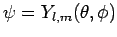. Calculate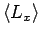and.

2. Find the eigenvalues and eigenfunctions (in terms of the anglesand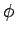) of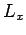.

3. Consider a beam of particles with. A measurement ofyields the result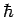. What values will be obtained by a subsequent measurement of, and with what probabilities? Repeat the calculation for the cases in which the measurement ofyields the results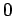and.

4. The Hamiltonian for an axially symmetric rotator is given by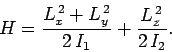What are the eigenvalues of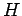?

Richard Fitzpatrick 2010-07-20